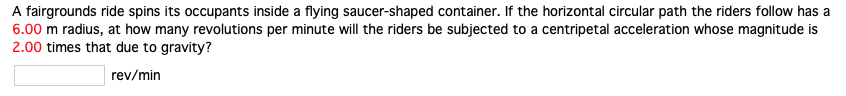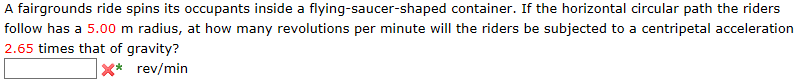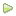Question

# A fairgrounds ride spins its occupants inside a flying saucer-shaped container. If the horizontal circular path...

A fairgrounds ride spins its occupants inside a flying saucer-shaped container. If the horizontal circular path the riders follow has a 8.00 m radius, at how many revolutions per minute will the riders be subjected to a centripetal acceleration whose magnitude is 2.45 times that due to gravity?

a = 2.45*g

= 2.45*9.8 m/s^2

= 24.01 m/s^2

= v^2/r = r*ω^2

so ω = sqrt(a/r) = sqrt(24.01/8.00) = 1.732 rad/s

= 1.732*60/2pi rev/min

= 16.54 rev/min

Kindly rate :)

#### Earn Coins

Coins can be redeemed for fabulous gifts.

Similar Homework Help Questions
• ### A fairgrounds ride spins its occupants inside a flying-saucer-shaped container. If the horizontal circular path the...

A fairgrounds ride spins its occupants inside a flying-saucer-shaped container. If the horizontal circular path the riders follow has a 8.00 m radius, at how many revolutions per minute will the riders be subjected to a centripetal acceleration 1.45 times that of gravity? rev/min

• ### A fairgrounds ride spins its occupants inside a flying-saucer-shaped container. If the horizontal circular path the...

A fairgrounds ride spins its occupants inside a flying-saucer-shaped container. If the horizontal circular path the riders follow has a 8.00 m radius, at how many revolutions per minute will the riders be subjected to a centripetal acceleration 2.85 times that of gravity? Answer in rev/min

• ### A fairgrounds ride spins its occupants inside a flying saucer-shaped container. If the horizontal circular path...A fairgrounds ride spins its occupants inside a flying saucer-shaped container. If the horizontal circular path the riders follow has a 6.00 m radius, at how many revolutions per minute will the riders be subjected to a centripetal acceleration whose magnitude is 2.00 times that due to gravity? rev/min

• ### A fairgrounds ride spins its occupants inside a flying-saucer-shaped container. If the horizontal circular path the...

A fairgrounds ride spins its occupants inside a flying-saucer-shaped container. If the horizontal circular path the riders follow has a 6.00 m radius, at how many revolutions per minute will the riders be subjected to a centripetal acceleration 2.95 times that of gravity?

• ### A fairgrounds ride spins its occupants inside a flying-saucer-shaped container. If the horizontal circular path the...

A fairgrounds ride spins its occupants inside a flying-saucer-shaped container. If the horizontal circular path the riders follow has a 7.00 m radius, at how many revolutions per minute will the riders be subjected to a centripetal acceleration 2.75 times that of gravity? rev/min

• ### A fairgrounds ride spins its occupants inside a flying-saucer-shaped container. If the horizontal circular path the...A fairgrounds ride spins its occupants inside a flying-saucer-shaped container. If the horizontal circular path the riders follow has a 5.00 m radius, at how many revolutions per minute will the riders be subjected to a centripetal acceleration 2.65 times that of gravity? x* rev/min

• ### A fairground ride spins its occupants inside a flying saucer-shaped container. If the horizontal circular path...

A fairground ride spins its occupants inside a flying saucer-shaped container. If the horizontal circular path the riders follow has a 6.3 m radius, if the riders is subjected to a centripetal acceleration 1.3 times that due to gravity, what is the spinning speed in unit of revolutions per minute? Use g = 9.8 m/s2.

• ### (8%) Problem 11: A fairground ride spins its occupants inside a flying saucer-shaped container. Randomized Variables...(8%) Problem 11: A fairground ride spins its occupants inside a flying saucer-shaped container. Randomized Variables r = 5.5 m ac = 1.55 times   If the horizontal circular path the riders follow has an 5.5 m radius, at how many revolutions per minute will the riders be subjected to a centripetal acceleration 1.55 times that due to gravity?

• ### A centrifuge is a device in which small container of material is rotated at a high speed on a circular path

A centrifuge is a device in which small container of material is rotated at a high speed on a circular path. Such a device is a used in medical laboratories, for instance, to cause the more dense red blood cells to settle through the less dense blood serum and collect at the bottom of the container. Suppose the centripetal acceleration of the sample is 6.60x10^3 times as large as the acceleration due to gravity. How many revolutions per minute is...

• ### Steve was subjected to accelerations in a horizontal "centrifuge". It is considered that such a device...

Steve was subjected to accelerations in a horizontal "centrifuge". It is considered that such a device has a radius of 5.0 m and can generate a centripetal acceleration equivalent to 7 times the average gravitational acceleration on Earth. a) For this maximum acceleration, what is the modulus of the astronaut's speed along the circular path? b) At how many revolutions per minute should the rotation occur to achieve such acceleration? c) What is the period of this rotation?